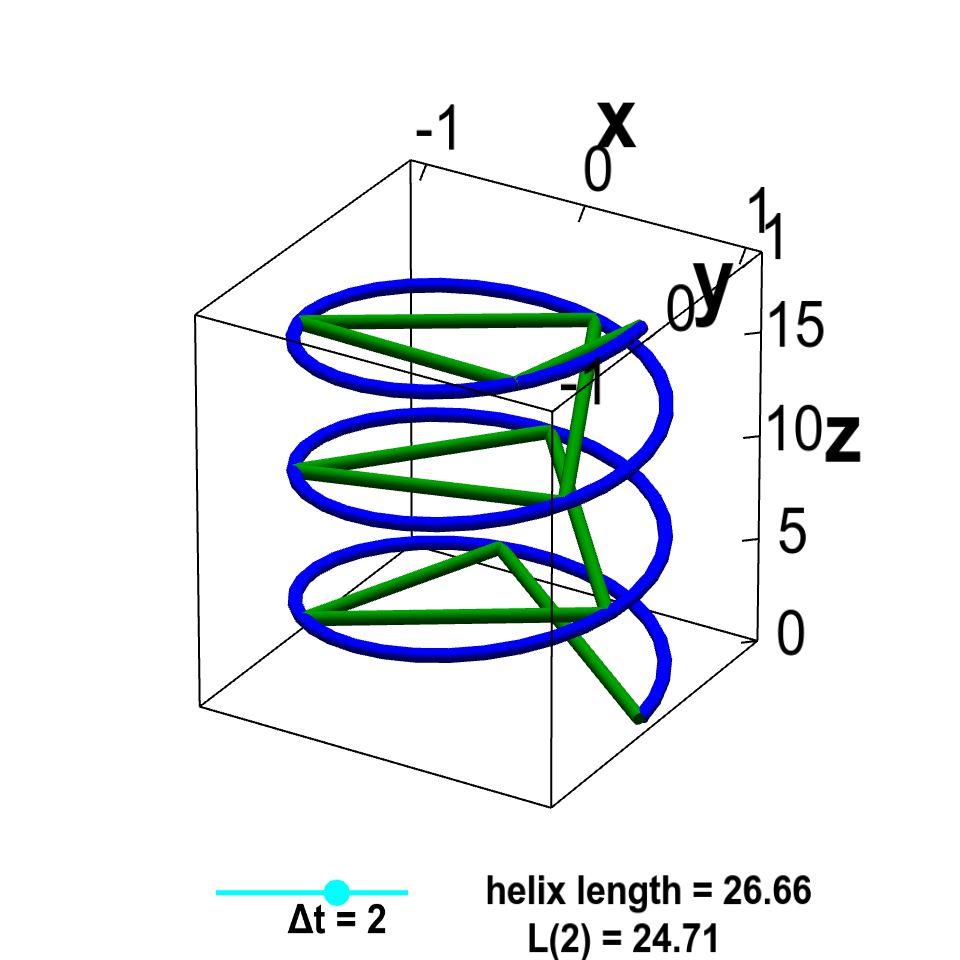# Math Insight

### Applet: Helix arc lengthThe vector-valued function $\dllp(t)=(\cos t, \sin t, t)$ parametrizes a helix, shown in blue. The green lines are line segments that approximate the helix. The discretization size of line segments $\Delta t$ can be changed by moving the cyan point on the slider. As $\Delta t \to 0$, the length $L(\Delta t)$ of the line segment approximation approaches the arc length of the helix from below.